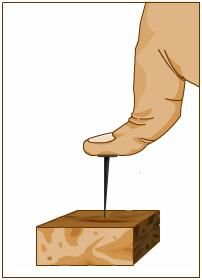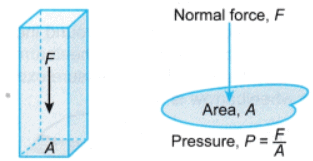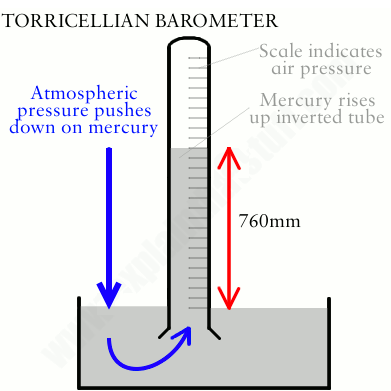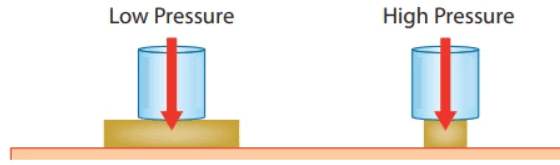Courses

# Thrust and Pressure Class 9 Notes | EduRev

## Class 9 : Thrust and Pressure Class 9 Notes | EduRev

The document Thrust and Pressure Class 9 Notes | EduRev is a part of Class 9 category.
All you need of Class 9 at this link: Class 9

Thrust

A force can be applied on a surface in any direction. When the force is applied on a surface in a direction normal (or perpendicular) to the surface, it is called thrust.DefinitionThe force acting normally on a surface is called thrust.

Thrust is a vector quantity.

Note:

•  A body when placed on a surface, exerts a thrust on the surface which is equal to its weight.
• The thrust is the same in whatsoever way the body is placed on the surface.

Unit of thrust

• It is measured in the units of force.
• The S.I. unit of thrust is newton (N) and C.G.S. unit is dyne.
1 N = 105 dyne
• The gravitational unit of thrust in M.K.S. system is kgf and in C.G.S. is gf.
1 kgf = 9.8 N and 1 gf = 980 dyneThrust and Pressure

Pressure

The effect of thrust depends on the area of the surface on which it acts. The effect of thrust is less if it acts on large area, while it is more if it acts on small area.

Example: If you stand on loose sand, your feet sink into the sand, but if you lie on that sand, your body does not sink into the sand. In both the cases the thrust exerted on the sand is the same (equal to your weight). But when you lie on sand, the thrust acts on a large area and when you stand, the same thrust acts on a small area.

The effect of thrust is expressed in terms of thrust per unit area. This quantity is called pressure.

DefinitionThe thrust or force on unit area of surface is called pressure.

If a thrust F acts on an area A, then

Pressure = Thrust/Area

or  P = F/ A

Pressure is a scalar quantity.

Units of Pressure

• The S.I. unit of pressure is Pascal (Pa) or newton per metre2 (N/m2).
• One pascal is the pressure exerted on a surface of area 1 m2 by a force of 1 N acting normally on it.
• The C.G.S. unit is dyne cm-2.
1 N/m2 = 10 dyne/cm2
• Other units of pressure are bar and millibar, where
1 bar = 105 N m-2 and 1 millibar = 10-3 bar = 102 N m-2
• The atmospheric pressure is generally expressed in terms of the height of the mercury column. At normal temperature and pressure, the barometric height is 0.76 m of Hg (or 76 cm of Hg or 760 mm of Hg) at sea level, which is taken as 1 atmosphere. Thus the atmospheric pressure is also expressed in a unit atmosphere (atm) where,
1 atmosphere (atm) = 0.76 m of Hg = 1.013 x 10Pa
• Sometimes we use torr as the unit of atmospheric pressure after the name of the scientist Torricelli where,
1 torr = 1 mm of HgFactors affecting the pressure: The pressure exerted on the surface depends on:

(1) the thrust

(2) the area on which the thrust is appliedNote:

The pressure on a surface is increased by making the area of surface small on which the thrust acts.

Example:

• The ends of nails or pins are made pointed so that large pressure is exerted at the pointed ends and they can be driven into with a less effort.
• The cutting tools also have either sharp or pointed edges, so that a smaller thrust may cause a greater pressure at the edges and cutting can be done with less effort.

Similarly, the pressure on a surface is reduced by making the area of surface large on which the thrust acts.

Example:

• Wide wooden sleepers are placed below the railway tracks so that the pressure exerted by the rails on the ground becomes less.
• The foundations of buildings are made wider than the walls, so that the pressure exerted by the building on the ground becomes less.

Offer running on EduRev: Apply code STAYHOME200 to get INR 200 off on our premium plan EduRev Infinity!

,

,

,

,

,

,

,

,

,

,

,

,

,

,

,

,

,

,

,

,

,

;